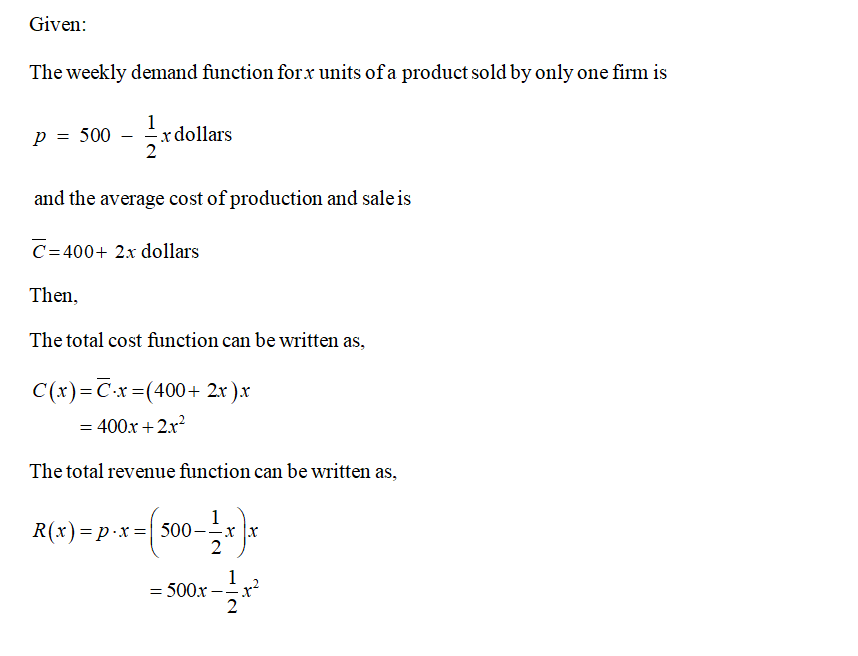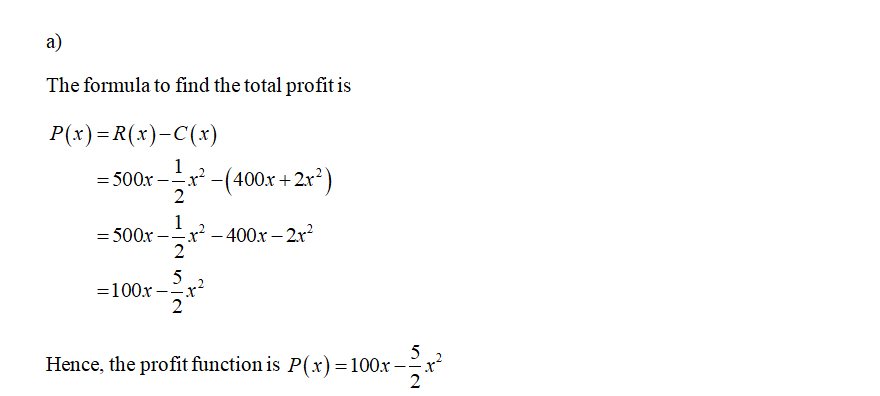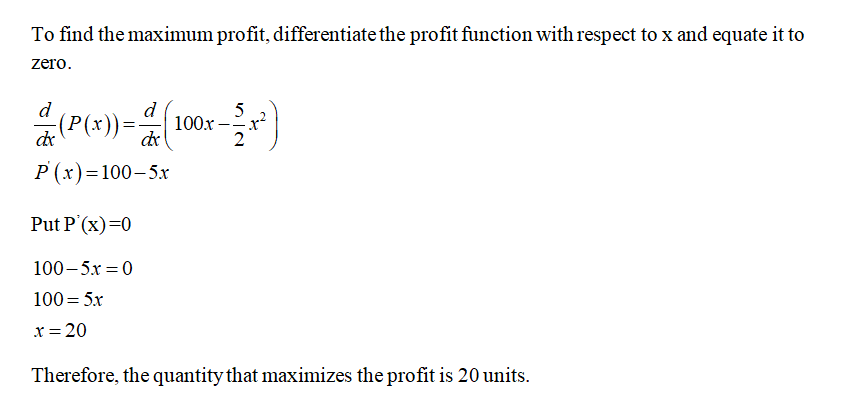# The weekly demand function for x units of a product sold by only one firm is p = 500 − 1/2x dollars, and the average cost of production and sale is C = 400 + 2x dollars.(a) Find the quantity that will maximize profit. units(b) Find the selling price at this optimal quantity.\$  per unit(c) What is the maximum profit?\$

Question
332 views

The weekly demand function for x units of a product sold by only one firm is

p = 500 − 1/2x dollars,

and the average cost of production and sale is

C = 400 + 2x dollars.
(a) Find the quantity that will maximize profit.
units

(b) Find the selling price at this optimal quantity.
\$  per unit

(c) What is the maximum profit?
check_circle

star
star
star
star
star
1 Rating
Step 1Step 2Step 3...

### Want to see the full answer?

See Solution

#### Want to see this answer and more?

Solutions are written by subject experts who are available 24/7. Questions are typically answered within 1 hour.*

See Solution
*Response times may vary by subject and question.
Tagged in